|

# 母亲节大礼放送丨把家扮美成为新工作?Ins上的全职妈妈都成功了!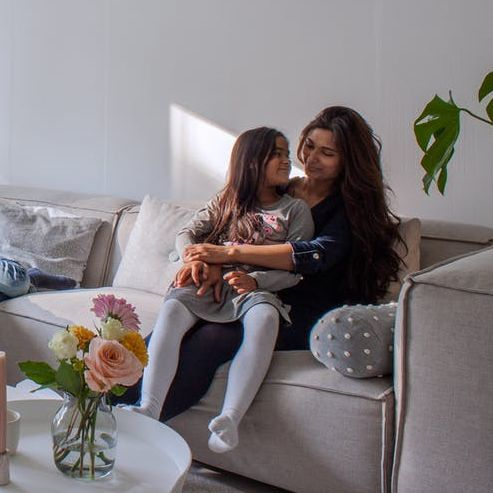▲当被问到她的标志性风格时，Sharita毫不犹豫地说:“轻木色、很多白色，再加一抹浪漫的粉色。我喜欢自然的材料，喜欢温暖而可爱的东西。”这实际上是Sharita一直以来的偏好。随着斯堪的纳维亚风的盛行，Sharita一直坚持的个人风格给她带来了意想不到的好处:她的家大受欢迎，最初在Instagram上分享家里照片的小消遣，现在变成了一份设计师的工作！▲沙发背景墙上一组有意思的字母组合装饰，Namaste在印地语里是表示问候的意思。▲窗边的铜钱草长得格外迷人，这个叶形如同规整圆形的品种，看上去格外时尚。可爱的木制猫头鹰是挪威设计师Lars Beller Fjetland的作品。▲Muuto在这个家庭中无处不在，电视柜、咖啡桌和落地灯都出自这个丹麦品牌。▲一个浅色的家和四个孩子，观者最多的问题无疑是:他们如何保持得如此干净?!“你知道我每周都要被问一次这个问题吗?”Sharita笑了。▲答案出奇的简单。“我们的室内一直是这样轻简的风格，所以孩子们都习惯了。从孩子出生开始，他们就会被要求遵守一些基本的规则，比如‘请在餐桌上吃饭’等等。另外，必须有足够的存储空间，沙发选择方便处理的面料。如果想要地毯，一定要选择质量好的单品，如果有人真的洒了（污渍），质量越好的地毯抗污能力越好。”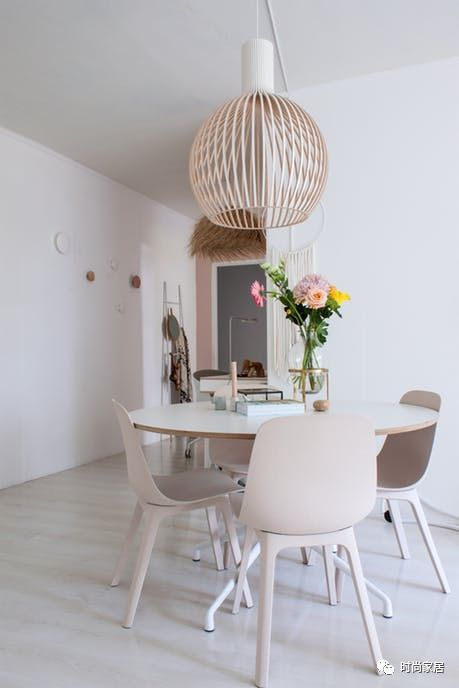▲来自宜家的碗状餐椅，材质是可再生木材和可再生塑料的混合物，好美貌，关注下国内的宜家商场是不是有上架……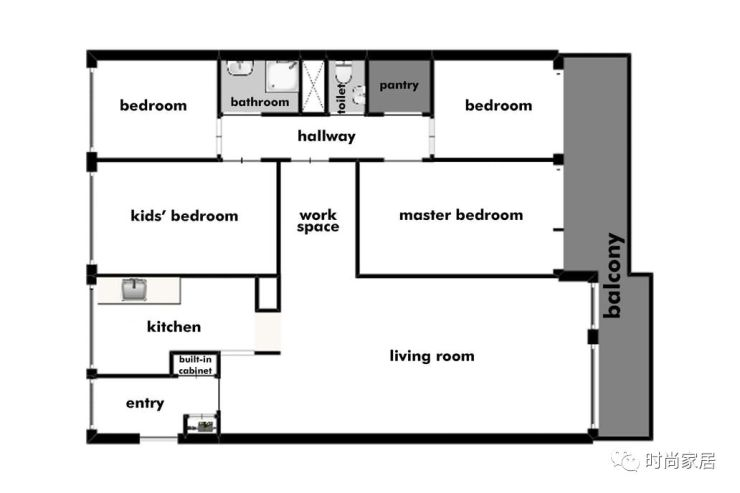▲户型图特地放在这里，是因为女主人工作室的位置比较特殊：设置在客厅与卧室间的走道上，看平面图，才能清楚明白这个布局。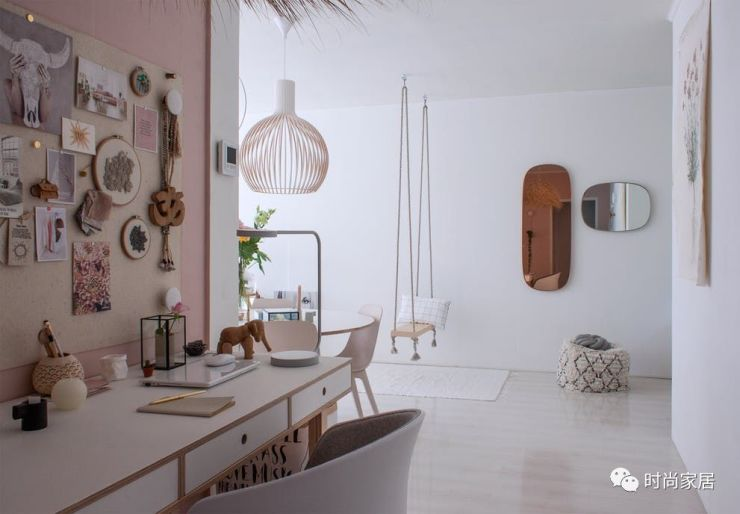▲“我的书桌靠近客厅。这是一个深思熟虑的决定。我可以相对独处，但同时我也能与孩子们一起分享空间，把他们留在我的视线里，不管是在餐桌上玩玩具，还是在沙发上看电影。”真是聪明的设计。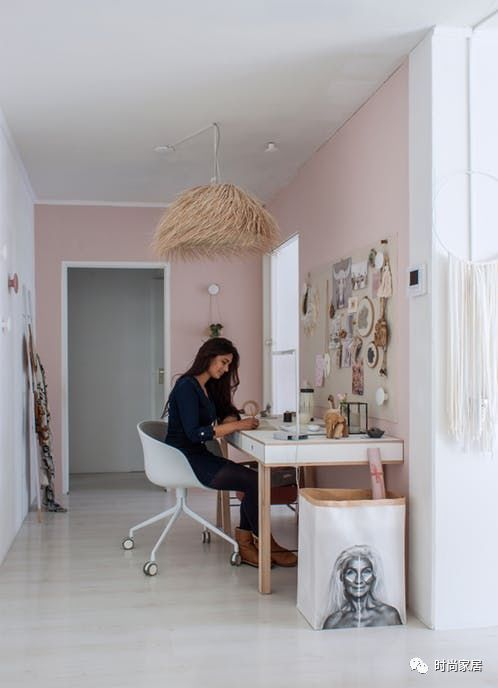▲Sharita选择了两面粉色的墙面，搭配一面采光很好的白墙，开放式工作室看上去精致而通透，利用得很棒！▲女主人最得意的DIY是她的备忘录板。“起初我想要一堵软木墙，因为我对天然材料很着迷。但事实证明，我对软木塞不感兴趣，它的色彩也太深。后来我找到这种完美的8毫米厚的厚毡子。有着柔软的色调，非常自然，正是我想要的。我剪出合适的尺寸，用一种特殊的胶水粘在墙上。它非常适合悬挂我所有可爱的东西，比如明信片和饰品。这就是灵感墙的伟大之处:你可以在不破坏墙壁的情况下，不断钉住和移除各种想法和主意。”▲简单的白色梯子提供了一种有趣的方式来收纳和展示漂亮的随手物品。▲小清新的厨房还是留有一点点遗憾。如果有机会，厨房可能会有所不同。全家人真的想要一个更开放的厨房，感觉就像是生活区域的延伸。Sharita 说：“加设黑框玻璃移门是不错的计划，谁知道呢，在不久的将来……”▲厨房的有孔钉板很有设计感，购自IKEA。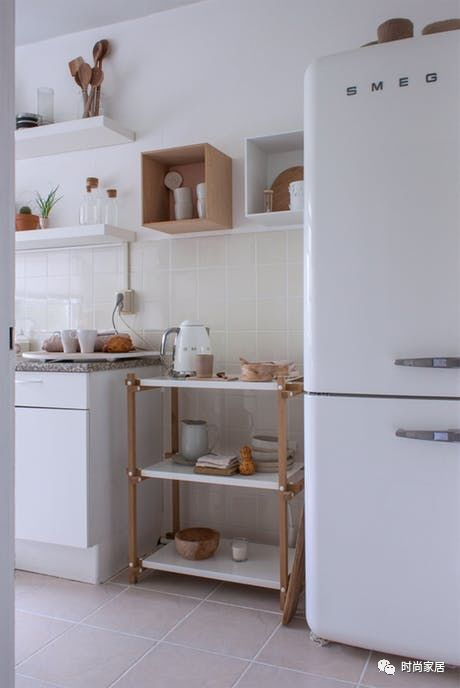▲来自HAY的置物架以及两只白色和木色收纳盒，搭配起来很好看。▲Sharita有一个让家变美的秘诀：必要地留空和留白。在油烟较少的料理台一边，用搁架替代厚重的收纳柜，空气和风都灵动起来。▲家里进门的门厅很小、很窄，这条狭长的换鞋长凳是宜家与HAY的合作款，因为喜欢HAY这款粉色的靠垫布料，Sharita询问了店员，发现她还可以定制与靠垫搭配的凳子，包括椅子的款式和尺寸的选择，“我觉得这把椅子是专门为我做的!”，这样的感觉还真特别。▲门厅设置大大的镜面，方便整理仪容，也不会感觉墙面太近、太局促。▲进入主卧室。在衣柜的侧面，有一个有趣的装饰。它贴了一块叫做布鲁克林Tins的墙纸，有着精美的图案和肌理。这是巴黎概念商店Merci和荷兰墙纸品牌NLXL的合作。后者以其独特的无重复壁纸而闻名。如果整面墙的使用预算太贵，选择一些小面积的壁纸装饰使用，也会是很好的体验。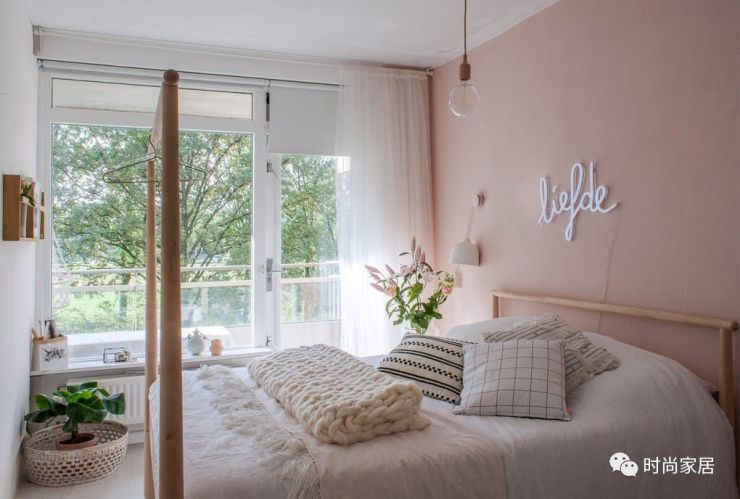▲这款宜家的桦木床可以让你选择用哪一头作为床头板。Shai和Sharita选择了低端，所以他们可以方便地把高端作为晚上挂衣服的地方。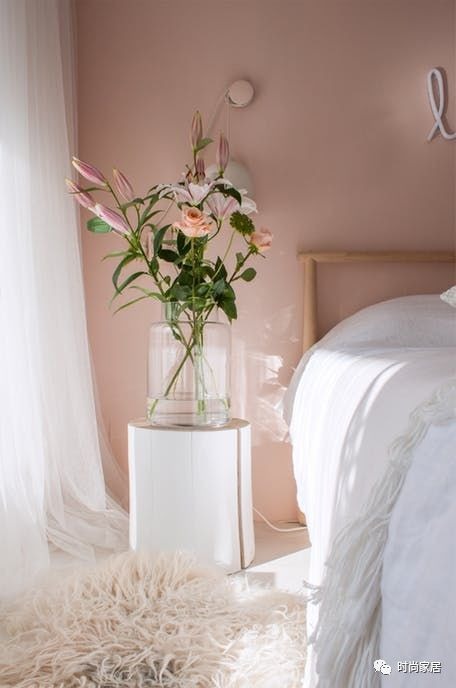▲“很多白色，一抹粉色，浪漫，轻木”，Sharita的标志性风格在房间里随处可见。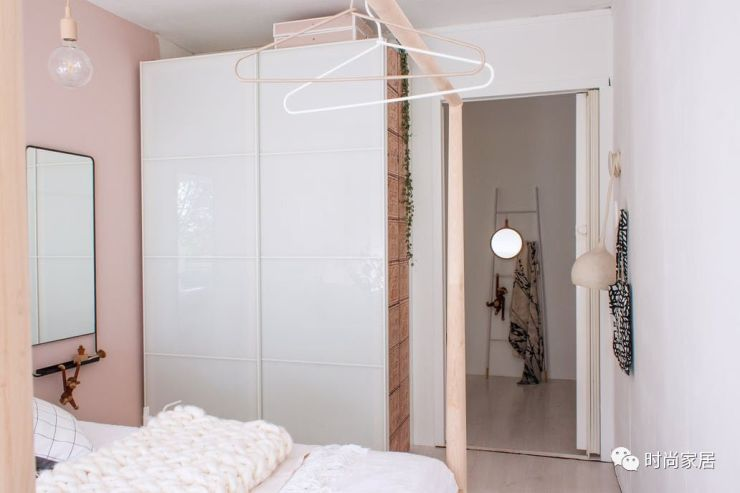▲卧室选择了一只精致的粉色吊灯，而门外工作室的布置与卧室风格也搭配合宜。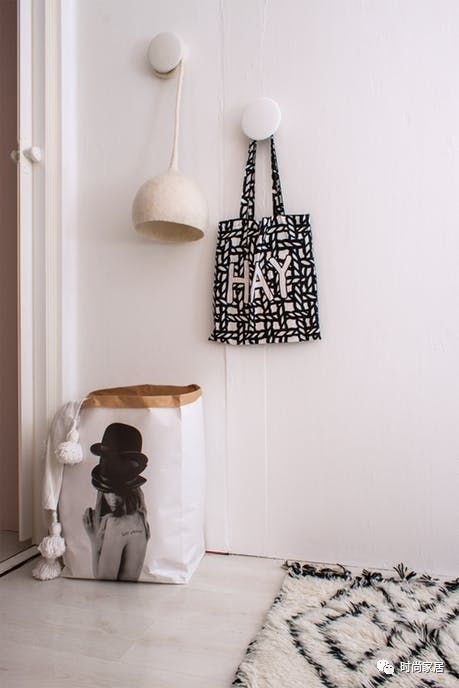▲不一定集中设置，圆点挂钩随意安置在各处墙面，好看也更实用。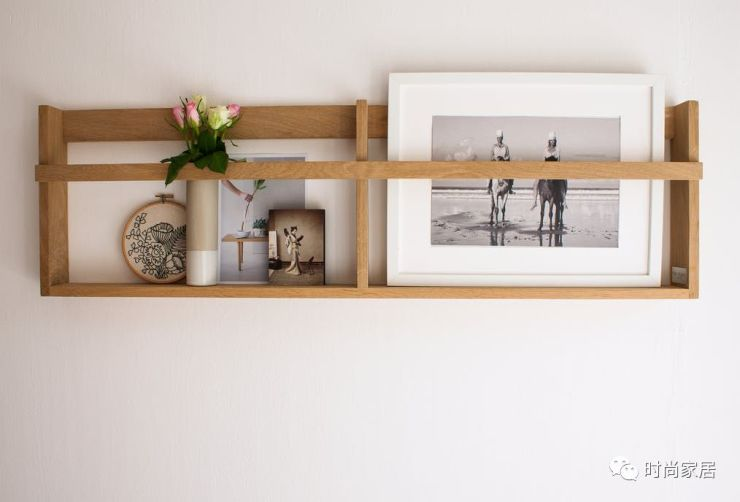▲卧室里木质搁架的小细节，用作摆放相框和装饰画，挺不错。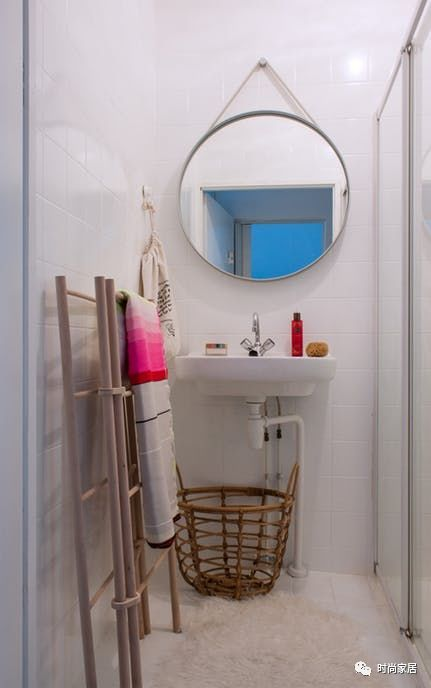▲全白色的浴室中添加一些天然材料和鲜艳的颜色，舒适又时髦。

▲年龄较小的两个孩子合用一间房间，采光很好。为了让他们感觉更放松和没有拘束，特别选择了做旧的白色砖墙效果的壁纸。▲Justin和Jasmijn很满意房间现在的样子。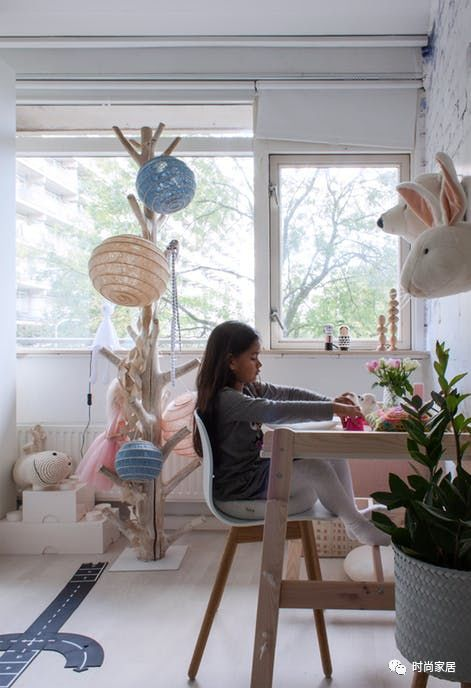▲Jasmijn特别喜欢学妈妈，坐在自己的小书桌上做一些可爱的小手工。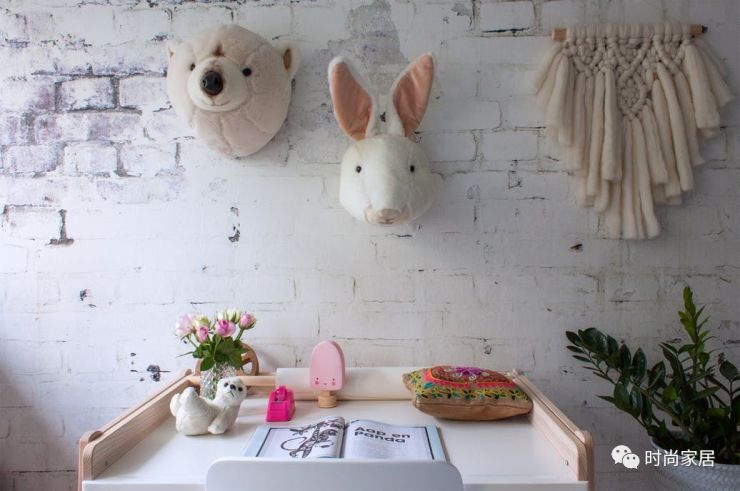▲被柔软而可爱的小物件环绕，生活如童话般美好。

`声明：本文由入驻焦点开放平台的作者撰写，除焦点官方账号外，观点仅代表作者本人，不代表焦点立场错误信息举报电话： 400-099-0099，邮箱：jubao@vip.sohu.com，或点此进行意见反馈，或点此进行举报投诉。`A B C D E F G H J K L M N P Q R S T W X Y Z
A - B - C - D - E
• A
• 鞍山
• 安庆
• 安阳
• 安顺
• 安康
• 澳门
• B
• 北京
• 保定
• 包头
• 巴彦淖尔
• 本溪
• 蚌埠
• 亳州
• 滨州
• 北海
• 百色
• 巴中
• 毕节
• 保山
• 宝鸡
• 白银
• 巴州
• C
• 承德
• 沧州
• 长治
• 赤峰
• 朝阳
• 长春
• 常州
• 滁州
• 池州
• 长沙
• 常德
• 郴州
• 潮州
• 崇左
• 重庆
• 成都
• 楚雄
• 昌都
• 慈溪
• 常熟
• D
• 大同
• 大连
• 丹东
• 大庆
• 东营
• 德州
• 东莞
• 德阳
• 达州
• 大理
• 德宏
• 定西
• 儋州
• 东平
• E
• 鄂尔多斯
• 鄂州
• 恩施
F - G - H - I - J
• F
• 抚顺
• 阜新
• 阜阳
• 福州
• 抚州
• 佛山
• 防城港
• G
• 赣州
• 广州
• 桂林
• 贵港
• 广元
• 广安
• 贵阳
• 固原
• H
• 邯郸
• 衡水
• 呼和浩特
• 呼伦贝尔
• 葫芦岛
• 哈尔滨
• 黑河
• 淮安
• 杭州
• 湖州
• 合肥
• 淮南
• 淮北
• 黄山
• 菏泽
• 鹤壁
• 黄石
• 黄冈
• 衡阳
• 怀化
• 惠州
• 河源
• 贺州
• 河池
• 海口
• 红河
• 汉中
• 海东
• 怀来
• I
• J
• 晋中
• 锦州
• 吉林
• 鸡西
• 佳木斯
• 嘉兴
• 金华
• 景德镇
• 九江
• 吉安
• 济南
• 济宁
• 焦作
• 荆门
• 荆州
• 江门
• 揭阳
• 金昌
• 酒泉
• 嘉峪关
K - L - M - N - P
• K
• 开封
• 昆明
• 昆山
• L
• 廊坊
• 临汾
• 辽阳
• 连云港
• 丽水
• 六安
• 龙岩
• 莱芜
• 临沂
• 聊城
• 洛阳
• 漯河
• 娄底
• 柳州
• 来宾
• 泸州
• 乐山
• 六盘水
• 丽江
• 临沧
• 拉萨
• 林芝
• 兰州
• 陇南
• M
• 牡丹江
• 马鞍山
• 茂名
• 梅州
• 绵阳
• 眉山
• N
• 南京
• 南通
• 宁波
• 南平
• 宁德
• 南昌
• 南阳
• 南宁
• 内江
• 南充
• P
• 盘锦
• 莆田
• 平顶山
• 濮阳
• 攀枝花
• 普洱
• 平凉
Q - R - S - T - W
• Q
• 秦皇岛
• 齐齐哈尔
• 衢州
• 泉州
• 青岛
• 清远
• 钦州
• 黔南
• 曲靖
• 庆阳
• R
• 日照
• 日喀则
• S
• 石家庄
• 沈阳
• 双鸭山
• 绥化
• 上海
• 苏州
• 宿迁
• 绍兴
• 宿州
• 三明
• 上饶
• 三门峡
• 商丘
• 十堰
• 随州
• 邵阳
• 韶关
• 深圳
• 汕头
• 汕尾
• 三亚
• 三沙
• 遂宁
• 山南
• 商洛
• 石嘴山
• T
• 天津
• 唐山
• 太原
• 通辽
• 铁岭
• 泰州
• 台州
• 铜陵
• 泰安
• 铜仁
• 铜川
• 天水
• 天门
• W
• 乌海
• 乌兰察布
• 无锡
• 温州
• 芜湖
• 潍坊
• 威海
• 武汉
• 梧州
• 渭南
• 武威
• 吴忠
• 乌鲁木齐
X - Y - Z
• X
• 邢台
• 徐州
• 宣城
• 厦门
• 新乡
• 许昌
• 信阳
• 襄阳
• 孝感
• 咸宁
• 湘潭
• 湘西
• 西双版纳
• 西安
• 咸阳
• 西宁
• 仙桃
• 西昌
• Y
• 运城
• 营口
• 盐城
• 扬州
• 鹰潭
• 宜春
• 烟台
• 宜昌
• 岳阳
• 益阳
• 永州
• 阳江
• 云浮
• 玉林
• 宜宾
• 雅安
• 玉溪
• 延安
• 榆林
• 银川
• Z
• 张家口
• 镇江
• 舟山
• 漳州
• 淄博
• 枣庄
• 郑州
• 周口
• 驻马店
• 株洲
• 张家界
• 珠海
• 湛江
• 肇庆
• 中山
• 自贡
• 资阳
• 遵义
• 昭通
• 张掖
• 中卫

1室1厅1厨1卫1阳台

1
2
3
4
5

0
1
2

1

1

0
1
2
3报名成功，资料已提交审核A B C D E F G H J K L M N P Q R S T W X Y Z
A - B - C - D - E
• A
• 鞍山
• 安庆
• 安阳
• 安顺
• 安康
• 澳门
• B
• 北京
• 保定
• 包头
• 巴彦淖尔
• 本溪
• 蚌埠
• 亳州
• 滨州
• 北海
• 百色
• 巴中
• 毕节
• 保山
• 宝鸡
• 白银
• 巴州
• C
• 承德
• 沧州
• 长治
• 赤峰
• 朝阳
• 长春
• 常州
• 滁州
• 池州
• 长沙
• 常德
• 郴州
• 潮州
• 崇左
• 重庆
• 成都
• 楚雄
• 昌都
• 慈溪
• 常熟
• D
• 大同
• 大连
• 丹东
• 大庆
• 东营
• 德州
• 东莞
• 德阳
• 达州
• 大理
• 德宏
• 定西
• 儋州
• 东平
• E
• 鄂尔多斯
• 鄂州
• 恩施
F - G - H - I - J
• F
• 抚顺
• 阜新
• 阜阳
• 福州
• 抚州
• 佛山
• 防城港
• G
• 赣州
• 广州
• 桂林
• 贵港
• 广元
• 广安
• 贵阳
• 固原
• H
• 邯郸
• 衡水
• 呼和浩特
• 呼伦贝尔
• 葫芦岛
• 哈尔滨
• 黑河
• 淮安
• 杭州
• 湖州
• 合肥
• 淮南
• 淮北
• 黄山
• 菏泽
• 鹤壁
• 黄石
• 黄冈
• 衡阳
• 怀化
• 惠州
• 河源
• 贺州
• 河池
• 海口
• 红河
• 汉中
• 海东
• 怀来
• I
• J
• 晋中
• 锦州
• 吉林
• 鸡西
• 佳木斯
• 嘉兴
• 金华
• 景德镇
• 九江
• 吉安
• 济南
• 济宁
• 焦作
• 荆门
• 荆州
• 江门
• 揭阳
• 金昌
• 酒泉
• 嘉峪关
K - L - M - N - P
• K
• 开封
• 昆明
• 昆山
• L
• 廊坊
• 临汾
• 辽阳
• 连云港
• 丽水
• 六安
• 龙岩
• 莱芜
• 临沂
• 聊城
• 洛阳
• 漯河
• 娄底
• 柳州
• 来宾
• 泸州
• 乐山
• 六盘水
• 丽江
• 临沧
• 拉萨
• 林芝
• 兰州
• 陇南
• M
• 牡丹江
• 马鞍山
• 茂名
• 梅州
• 绵阳
• 眉山
• N
• 南京
• 南通
• 宁波
• 南平
• 宁德
• 南昌
• 南阳
• 南宁
• 内江
• 南充
• P
• 盘锦
• 莆田
• 平顶山
• 濮阳
• 攀枝花
• 普洱
• 平凉
Q - R - S - T - W
• Q
• 秦皇岛
• 齐齐哈尔
• 衢州
• 泉州
• 青岛
• 清远
• 钦州
• 黔南
• 曲靖
• 庆阳
• R
• 日照
• 日喀则
• S
• 石家庄
• 沈阳
• 双鸭山
• 绥化
• 上海
• 苏州
• 宿迁
• 绍兴
• 宿州
• 三明
• 上饶
• 三门峡
• 商丘
• 十堰
• 随州
• 邵阳
• 韶关
• 深圳
• 汕头
• 汕尾
• 三亚
• 三沙
• 遂宁
• 山南
• 商洛
• 石嘴山
• T
• 天津
• 唐山
• 太原
• 通辽
• 铁岭
• 泰州
• 台州
• 铜陵
• 泰安
• 铜仁
• 铜川
• 天水
• 天门
• W
• 乌海
• 乌兰察布
• 无锡
• 温州
• 芜湖
• 潍坊
• 威海
• 武汉
• 梧州
• 渭南
• 武威
• 吴忠
• 乌鲁木齐
X - Y - Z
• X
• 邢台
• 徐州
• 宣城
• 厦门
• 新乡
• 许昌
• 信阳
• 襄阳
• 孝感
• 咸宁
• 湘潭
• 湘西
• 西双版纳
• 西安
• 咸阳
• 西宁
• 仙桃
• 西昌
• Y
• 运城
• 营口
• 盐城
• 扬州
• 鹰潭
• 宜春
• 烟台
• 宜昌
• 岳阳
• 益阳
• 永州
• 阳江
• 云浮
• 玉林
• 宜宾
• 雅安
• 玉溪
• 延安
• 榆林
• 银川
• Z
• 张家口
• 镇江
• 舟山
• 漳州
• 淄博
• 枣庄
• 郑州
• 周口
• 驻马店
• 株洲
• 张家界
• 珠海
• 湛江
• 肇庆
• 中山
• 自贡
• 资阳
• 遵义
• 昭通
• 张掖
• 中卫• 手机• 分享
• 设计
免费设计
• 计算器
装修计算器
• 入驻
合作入驻
• 联系
联系我们
• 置顶
返回顶部